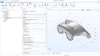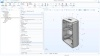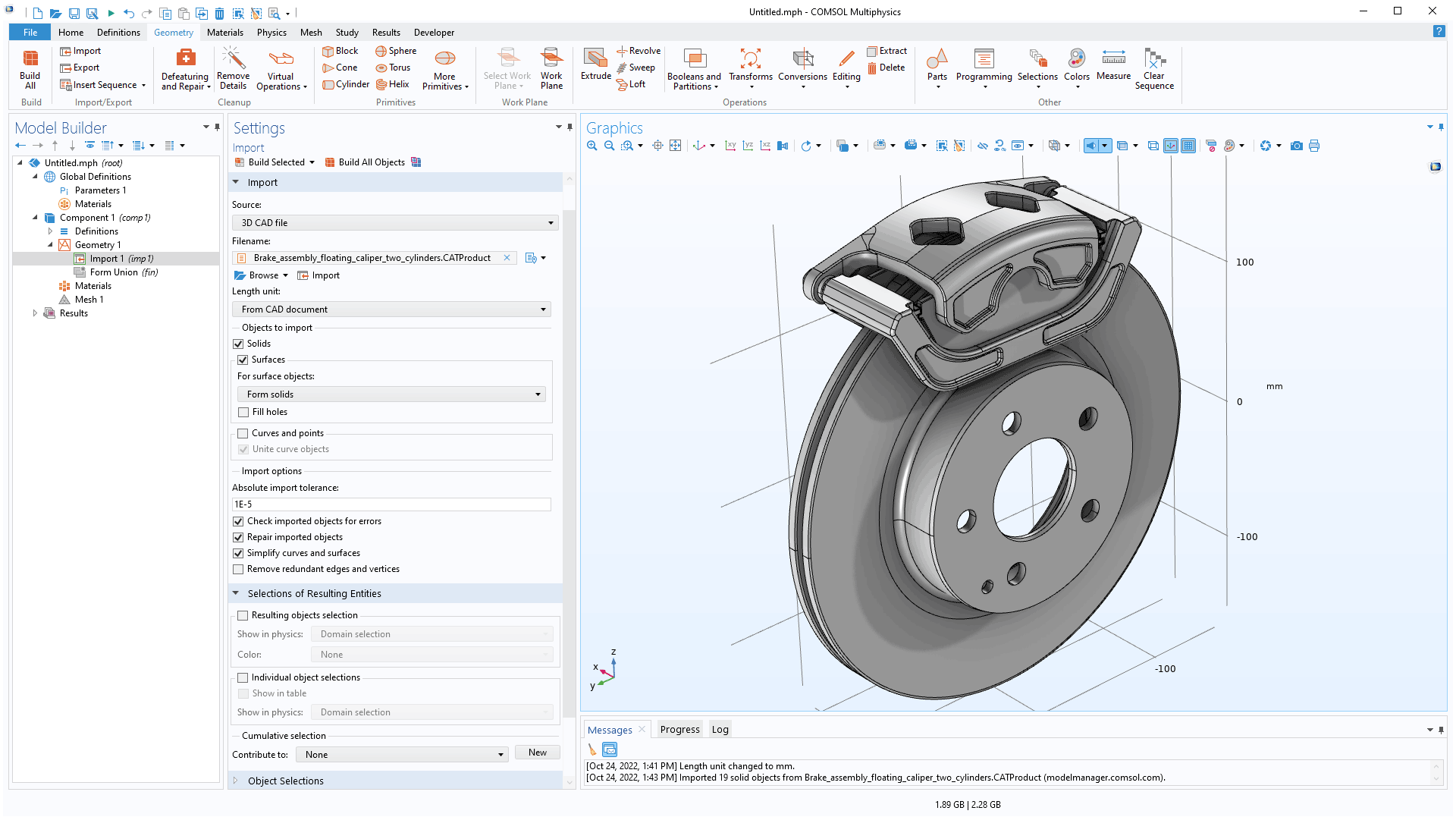# COMSOL Multiphysics® 6.1 Release Highlights

support@comsol.com

For users of the Design Module, version 6.1 introduces two new operations and one tutorial model. Read more about these updates below.

### Offset Faces

With the new Offset Faces geometry operation, you can offset faces on 3D geometry objects in the normal direction. In addition to being a useful tool for editing 3D geometries, this operation makes it possible to parameterize geometric features on geometry objects imported from 3D CAD files. In MEMS applications, you can apply this operation to efficiently create 3D geometry that emulates the deposition of material onto a nonflat surface. The Offset Faces operation is exemplified in the new Normal Modes of a Biased Resonator — 3D Geometry and Optimization of an Imported Bracket Geometry Tutorial Series models.Using the new Offset Faces geometry operation to enlarge the holes of the geometry of a brake caliper, imported from a STEP file.

### Transform Faces

The new Transform Faces operation applies a linear transformation — which can consist of displacement, rotation, and isotropic scaling on a selection of faces — on 3D geometry objects. This functionality is especially useful when modifying geometry models imported from 3D CAD files. For example, the Transform Faces operation can be used to parameterize geometric features of the imported objects, sweep over these parameters, and optimize the geometry using the Optimization Module. You can view this new feature in the Optimization of an Imported Bracket Geometry Tutorial Series model.The Transform Faces operation is used to move a mounting feature on the geometry of an enclosure, imported from a STEP file. A coordinate system is added to define the direction of the displacement along a geometry edge.

### Simplifying Curves and Surfaces

The import settings for 3D CAD files now include a Simplify curves and surfaces option, which can be used to simplify the mathematical representation of the imported geometric entities. For example, spline curves can be converted to dedicated straight or circular curves. Importing objects with this option can improve the performance and reliability of downstream geometric operations.Importing a brake assembly model using the new Simplify curves and surfaces option converts, where applicable, the underlying mathematical representation of edges and faces to a simpler analytical form.